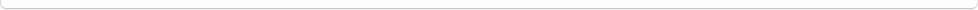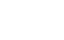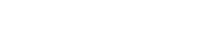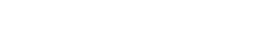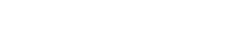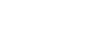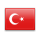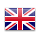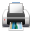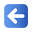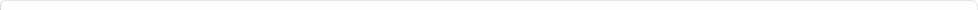Description of Individual Course Units
 Course Unit Code Course Unit Title Type of Course Unit Year of Study Semester Number of ECTS Credits İŞL-23-111 Elective 1 1 6
Level of Course Unit
Second Cycle
Objectives of the Course
To provide information about different models and decision analysis techniques for making decisions under certainty and uncertainty by using concepts such as statistical decision theory, utility theory, decision trees, Bayes theorem.
Name of Lecturer(s)
Learning Outcomes
 1 1. To know the basic concepts of statistical decision theory.; 2 . To be able to define the decision making problem.; 3. To be able to solve cost structured decision problems; 4. To know the concepts and rules of game theory.; 5. To be able to apply the analysis of decision making under uncertainty and risk. 6 . To be able to apply decision tree analysis; 7. To be able to use sample information while making statistical decisions; 8 . Using Bayes' theorem while making statistical decisions; 9 . To be able to apply Markov analysis; 10. To know the multi-criteria decision making methods.; 2 1 To know the basic concepts of statistical decision theory.; 2 To be able to define the decision making problem.; 3 To be able to solve cost structured decision problems; 4 To know the concepts and rules of game theory.; 5 To be able to apply the analysis of decision making under uncertainty and risk. 6 To be able to apply decision tree analysis; 7 To be able to use sample information while making statistical decisions; 8 Using Bayes' theorem while making statistical decisions; 9 To be able to apply Markov analysis; 10 To know the multi-criteria decision making methods.;
Mode of Delivery
Daytime Class
Prerequisites and co-requisities
none
Recommended Optional Programme Components
none
Course Contents
1 Existence of Utility Function 2. A Game and _Testing Statistical Hypotheses 3. : Properties and Expansion of Utility Function II 4. Convex Clusters I 5. Utility Function and _Statistics: Distribution Parameters 6. Decision Making Under the Uncertainty of Natural Situations I 7. The Minimax _Principle in the Uncertainty of Nature 8. Bayesian Principle in the Uncertainty of Nature 9. Admissibility and Decision-Making Principles 10. Selection of Risk Function and Decision Function 11. Postfinal Distribution and Expected Postpartum Loss 12. Parameter Estimation as a Decision-Making Problem 13. Obtaining the Bayesian Estimator with Final Losses 14. Utility Function and _Statistics: Distribution Parameters
Weekly Detailed Course Contents
 Week Theoretical Practice Laboratory 1 1. Existence of Utility Function 2 2. A Game and _Testing Statistical Hypotheses 3 3. Properties and Extension of Utility Function I 4 4. Convex Clusters 5 5. Utility Function and _Statistics: Distribution Parameters 6 6. Decision Making Under the Uncertainty of Natural Situations I 7 7. The Minimax _Principle in the Uncertainty of Nature 8 8. Bayesian Principle in the Uncertainty of Nature 9 9. Admissibility and Decision-Making Principles 10 10. Selection of Risk Function and Decision Function 11 11. Postfinal Distribution and Expected Postpartum Loss 12 12. Parameter Estimation as a Decision-Making Problem 13 13. Obtaining the Bayesian Estimator with Final Losses 14 14. Utility Function and _Statistics: Distribution Parameters
1.James Berger, Statistical Decision Theory and Bayesian Analysis, Springer-Verlag, 1980. 2.Mustafa Aytaç, Necmi Gürsakal (editörler), Karar Verme, Dora Yayınları, 2015.
Planned Learning Activities and Teaching Methods
Assessment Methods and Criteria
 Term (or Year) Learning Activities Quantity Weight Midterm Examination 1 100 SUM 100 End Of Term (or Year) Learning Activities Quantity Weight Final Examination 1 100 SUM 100 Term (or Year) Learning Activities 40 End Of Term (or Year) Learning Activities 60 SUM 100
Language of Instruction
Work Placement(s)
none